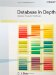# Appendix A. A Little Bit of Logic

 In Chapter 1, I mentioned that there's an alternative to the relational algebra called the relational calculus. Relational calculus is essentially an applied form of predicate calculus, tailored to the needs of relational databases. Queries and constraints, for example, can be formulated using relational calculus instead of relational algebra; indeed, sometimes it's easier to come up with a calculus formulation (on the other hand, sometimes the opposite is true). And it's desirable, in my opinion, for database professionals to have at least a nodding acquaintance with the basic concepts of the calculus as well as the algebra; hence this appendix, which offers a brief and very informal introduction to such matters. By the way, it follows from the preceding paragraph that a relational language can be based on either the algebra or the calculus. So which is SQL based on? The answer, regrettably, is partly both and partly neither. When it was first designed, SQL was specifically intended to be different from both the algebra and the calculus; indeed, such a goal was the prime motivation for the introduction of the SQL "IN subquery" construct. As time went by, however, it turned out that certain features of both the algebra and the calculus were needed after all, and the language grew to accommodate them. The situation today is thus that some aspects of SQL are "algebra-like," some are "calculus-like," and some are neither.Database in Depth: Relational Theory for Practitioners
ISBN: 0596100124
EAN: 2147483647
Year: 2006
Pages: 127
Authors: C.J. Date

Similar book on Amazon